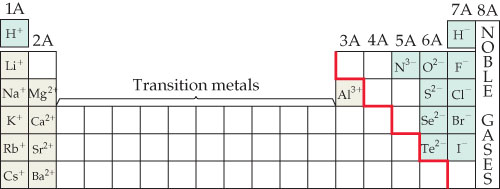# Problem: Predictable charges of some common ions. Notice that the red stepped line that divides metals from nonmetals also separates cations from anions. Hydrogen forms both 1+ and 1- ions.The most common ions for silver, zinc, and scandium are Ag+, Zn2+, and Sc3+. Which of these ions have the same number of electrons as a noble-gas element?

###### FREE Expert Solution

We’re being asked to determine the ion that has the same number of electrons as a number of a noble-gas element

Before we can do that, let’s first determine the total number of electrons in a neutral silver, zinc, and scandium atoms by writing its electron configuration.

90% (247 ratings)###### Problem DetailsPredictable charges of some common ions. Notice that the red stepped line that divides metals from nonmetals also separates cations from anions. Hydrogen forms both 1+ and 1- ions.

The most common ions for silver, zinc, and scandium are Ag+, Zn2+, and Sc3+. Which of these ions have the same number of electrons as a noble-gas element?